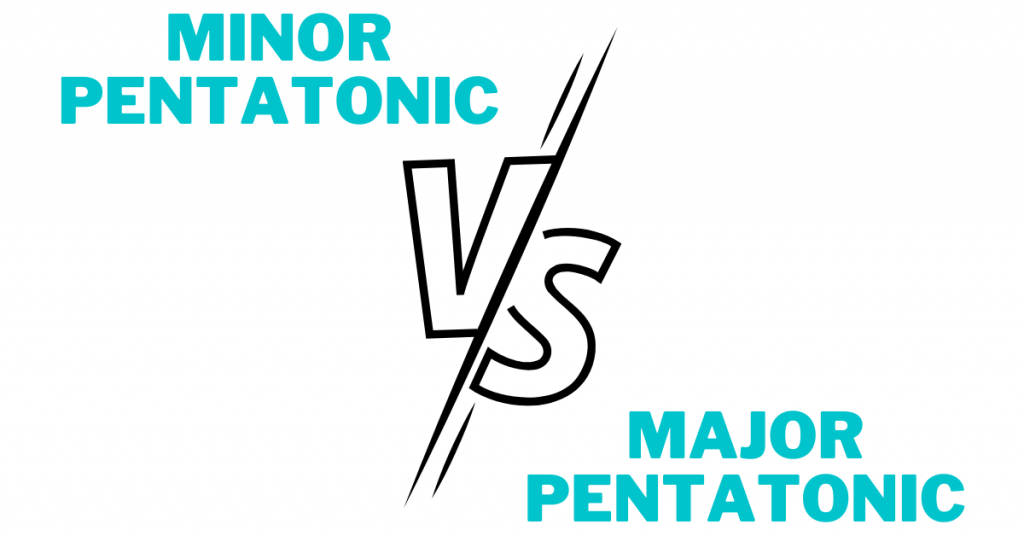# Difference Between Major and Minor Pentatonic ScalesLearning pentatonic scales can be confusing when you start to see words thrown around like “major” and “minor” all the time.

So, let’s get rid of your confusion.

There are two ways to explain the difference between major and minor pentatonic scales.

## 1. Derivative Pentatonic Theory

Derivative pentatonic theory is where we take a group of notes and derive our scales from those same notes.

For example, the A minor pentatonic scale and C major pentatonic scale contain the exact same notes!

A-C-D-E-G

#### Notes in C Major Pentatonic

C-D-E-G-A

The scale name is determined by the context of those notes.

For example, if you’re using these notes over a C major chord, you are implying C major pentatonic.

If you’re playing these notes over an A minor chord, you are implying A minor pentatonic.

## Derivative pentatonic examples

### A Minor Pentatonic

Here you have A Minor Pentatonic shape 1.

The root note highlighted is A, so you know it’s minor pentatonic.

### C Major Pentatonic

Here you have C major pentatonic shape 1.

The root note highlighted is C, so you know it’s major pentatonic.

## 2. Parallel Pentatonic Theory

Parallel pentatonic theory is where we look at how a scale relates back to it’s root note.

This is done by building the scale using a formula starting from the root note (tonic).

Let’s look at the scale formulas for major and minor pentatonic scales.

1-2-3-5-6

### Minor pentatonic scale formula

1-b3-4-5-b7

Let’s use A minor pentatonic and A major pentatonic for examples.

## Summary: Difference between major and minor pentatonic scales

To summarize, the difference between major and minor pentatonic scales can be determined by the derived group of notes, or by the formula related to the tonic.

Understanding both parallel and derivative pentatonic theory is the key to understanding major and minor pentatonic scales as a whole!

After learning those, I suggest getting started with the blues scale.